# Suppose you have a circular cross sectional wire with radius R, a resistivity of ρ, and...

Suppose you have a circular cross sectional wire with radius R, a resistivity of ρ, and that is carrying a uniformly distributed current of I(t) = I0 cos(ωt) (notice it changes in time).

a) Find the electric field that is generating this current using the proper form of Ohm’s Law.

b) Find the magnetic field outside of the wire that is generated by the current and the time changing electric field combined.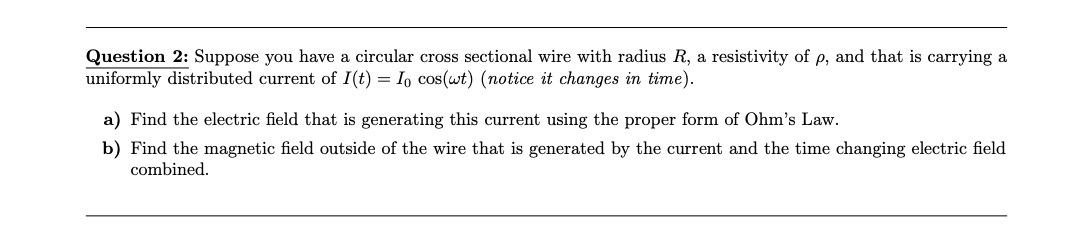Question 2: Suppose you have a circular cross sectional wire with radius R, a resistivity of p, and that is carrying a uniformly distributed current of I(t) = 1, cos(wt) (notice it changes in time). a) Find the electric field that is generating this current using the proper form of Ohm's Law. b) Find the magnetic field outside of the wire that is generated by the current and the time changing electric field combined.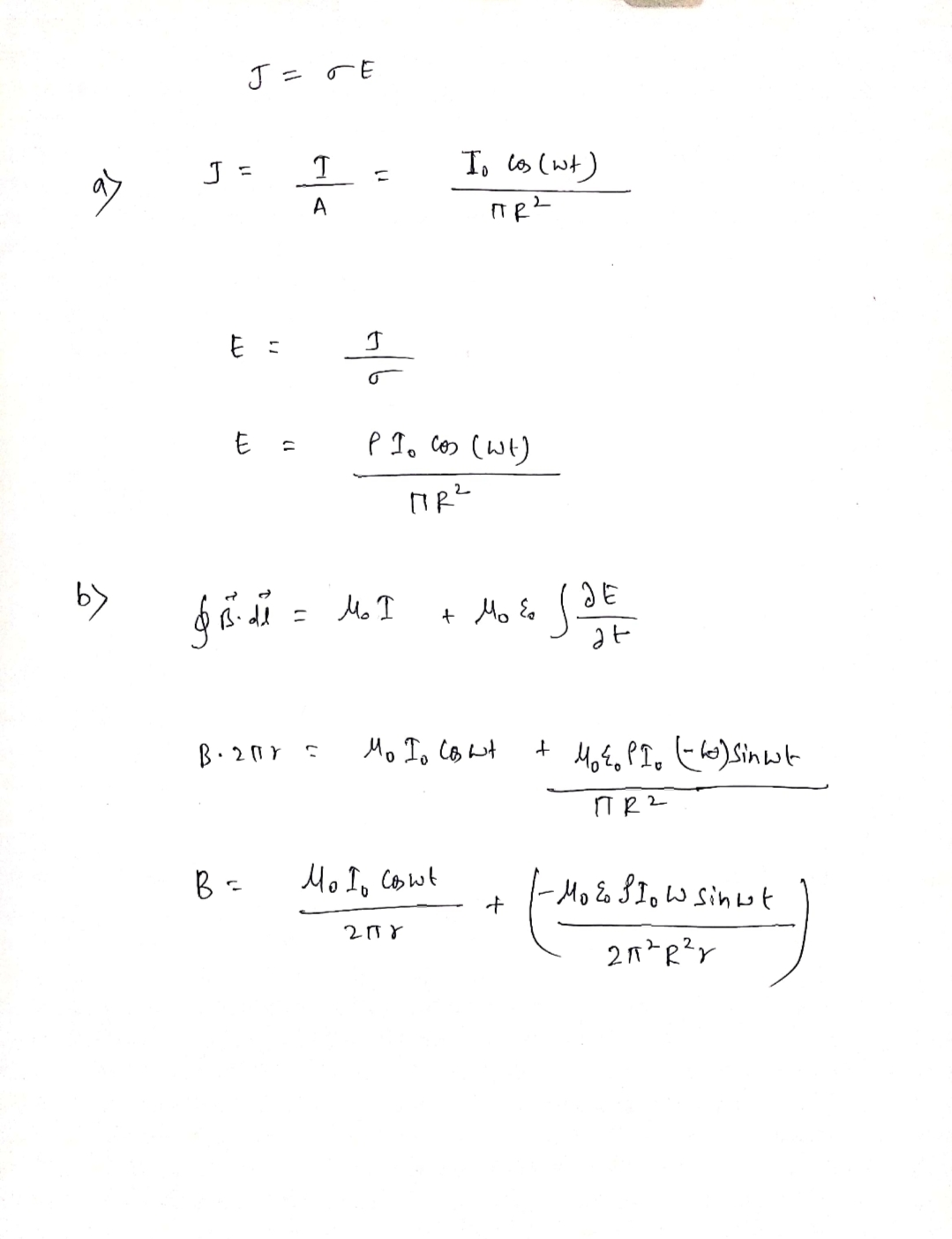##### Add Answer of: Suppose you have a circular cross sectional wire with radius R, a resistivity of ρ, and...
Similar Homework Help Questions
• ### A long, straight, copper wire with a circular cross-sectional are of 1.5mmm^2 carries a current of...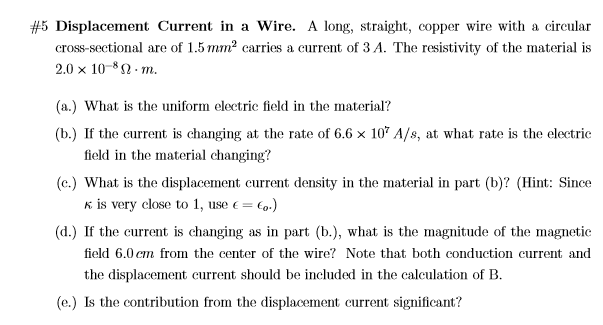A long, straight, copper wire with a circular cross-sectional are of 1.5mmm^2 carries a current of 3 A . #5 Displacement Current in a wire. A long, straight, copper wire with a circular are of 1.5 mm2 carries a current of 3 A. The resistivity of the material is cross-sectional are of 1.5mm2crries a current of 3A. The resistivity of the material is 2.0 × 10-8 Ω·m. (a.) What is the uniform electric field in the material? (b) If the...

• ### Magnetism urgent 3. (20 points) A very long C'nfinite") solenoid has cross sectional area A. There...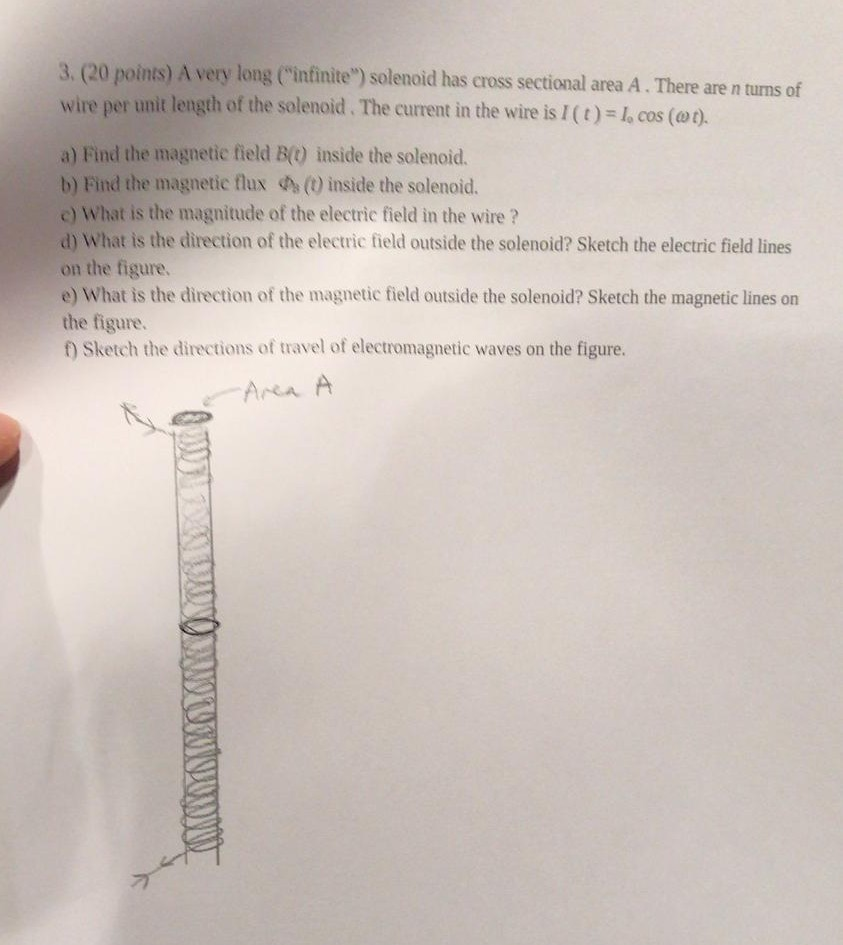Magnetism urgent 3. (20 points) A very long C'nfinite") solenoid has cross sectional area A. There are n turns of wire per unit length of the solendi d . The current in the wire is 1 ( t ) cos (ω t). a) Find the magnetic field B() inside the solenoid. b) Find the magnetic flux inside the solenoid. c) What is the magnitude of the electric field in the wire? d) What is the direction of the electric field...

• ### Copper Wire cross-sectional area

A copper wire with a cross-sectional area of 2.25 x 10^-7 m^2 carries a current of 7.50 x 10^-3 A. The number of charge carriers per volume for copper is 8.47 x 10^28electrons/m^3, and the resistivity of copper is 1.69 x 10^-8 ohm meter.a) What is the drift velocity of the electrons?b) What is the electric field in the wire?c) For the conduction electrons, what is the average time between collisions?

• ### 11. An aluminum wire with a square cross-section 2 mm on a side is carrying a current of 1.5 amps. Aluminum has a resistivity of 2.69 x 10* ohm-cm and an atormic volume of 10 cc/mol. Assuming alum...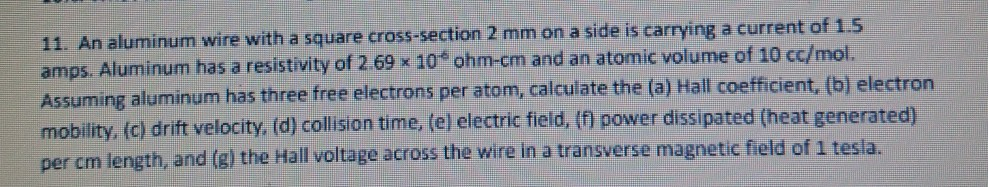11. An aluminum wire with a square cross-section 2 mm on a side is carrying a current of 1.5 amps. Aluminum has a resistivity of 2.69 x 10* ohm-cm and an atormic volume of 10 cc/mol. Assuming aluminum has three free electrons per atom, calculate the (a) Hall coeffcient, (b) electron mobility, (c) drift velocity, d) collision time. (e) electric field, (f) power dissipated (heat generated) per cm length, and (g) the Hall voltage across the wire in a transverse...

• ### A wire of radius 0.7 cm carries a current of 101 A that is uniformly distributed...

A wire of radius 0.7 cm carries a current of 101 A that is uniformly distributed over its cross-sectional area. Find the magnetic field B at a distance of 0.09 cm from the center of the wire.

• ### (a) (10 marks] A straight wire along the ź direction with a circular cross-section of radius...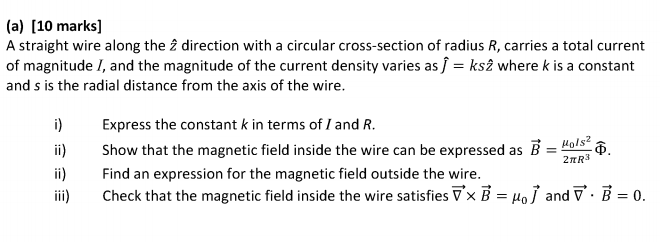(a) (10 marks] A straight wire along the ź direction with a circular cross-section of radius R, carries a total current of magnitudel, and the magnitude of the current density varies as I = ks 2 where k is a constant and s is the radial distance from the axis of the wire. i) Express the constant k in terms of I and R. Show that the magnetic field inside the wire can be expressed as B = 80. Find...

• ### nand a cross sectional area of 0.270 mm2. If the resistivity of copper is 1.70 x...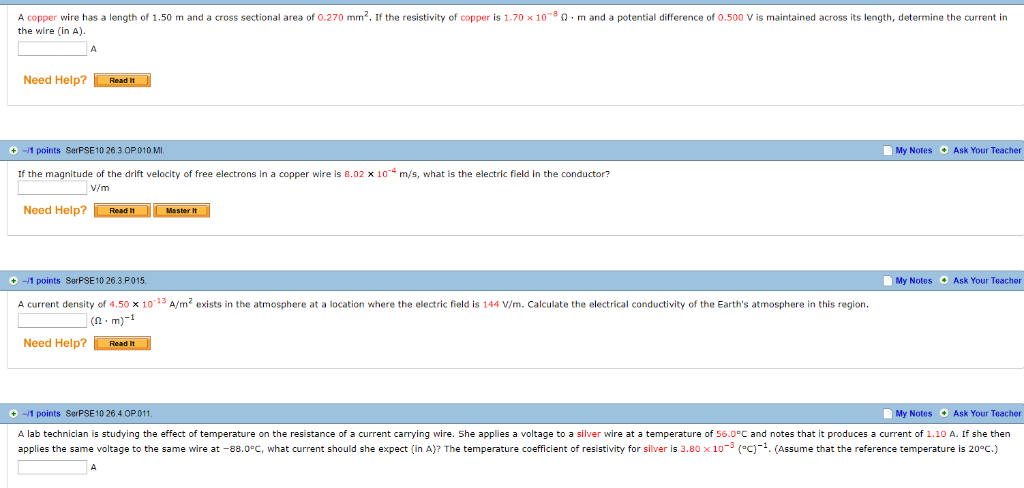nand a cross sectional area of 0.270 mm2. If the resistivity of copper is 1.70 x 10 m and a potential difference of 0.500 V is maintained across its lenqth, determine the current in has a length of 1.50 the wire (In A) Need Help? Read It -1 points SerPSE10 26.3.0P010 M Ask Your Teacher My Notes f the magnitude of the drift velocity of free electrons in a copper wire i 10 m/s. what the electric field in the...

• ### A circular loop of wire of resistance - 0.500 and radius 8.10 cm is in a...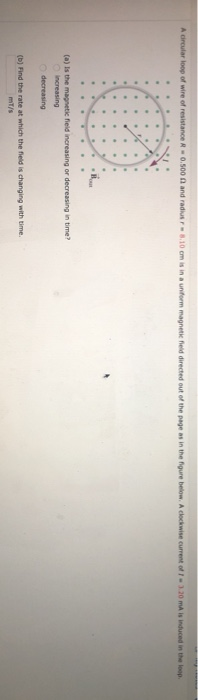A circular loop of wire of resistance - 0.500 and radius 8.10 cm is in a uniform magnetic field directed out of the page as in the figure below. A clockwise current of 120 mA is induced in the loop. (a) is the magnetic field increasing or decreasing in time? decreasing (b) Find the rate at which the field is changing with time.

• ### There is a circular ring of wire. It has a radius α that carries a current in a counter clockwise...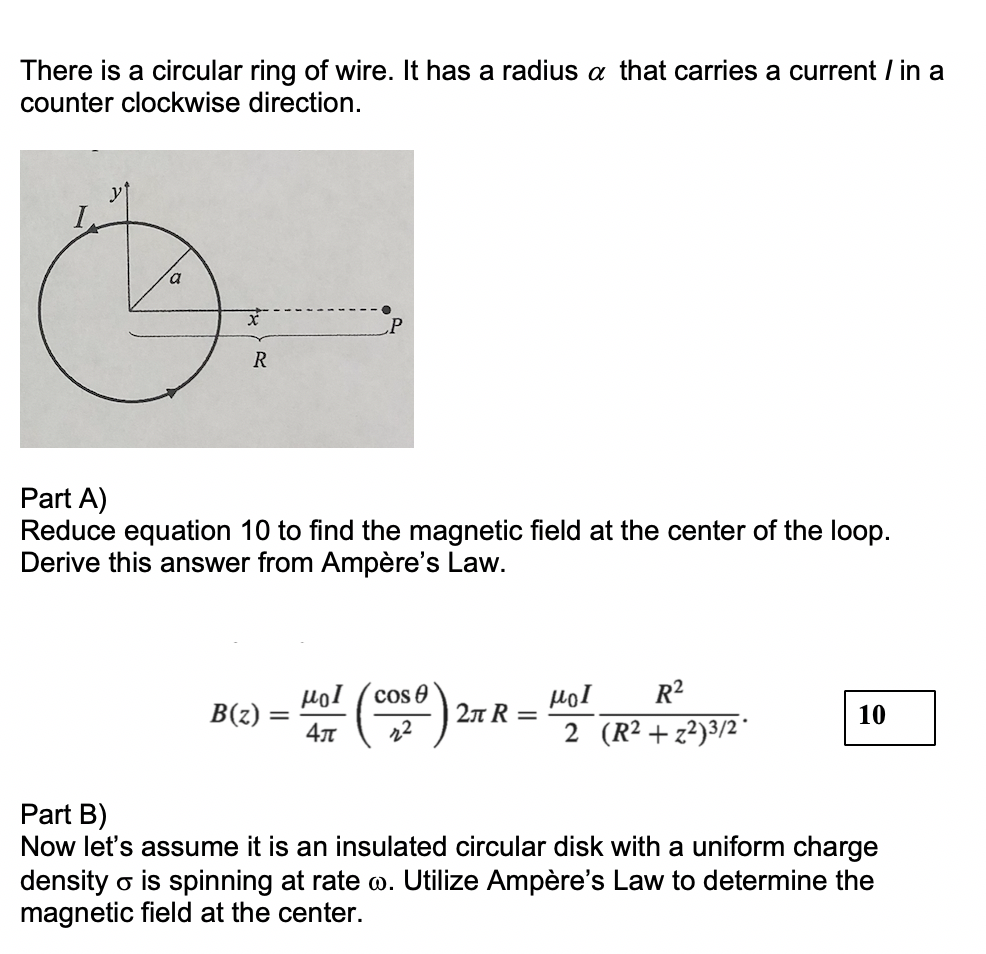There is a circular ring of wire. It has a radius α that carries a current in a counter clockwise direction L.P Part A) Reduce equation 10 to find the magnetic field at the center of the loop. Derive this answer from Ampère's Law Ho R2 401 (cos θ 10 B(z) Part B) Now let's assume it is an insulated circular disk with a uniform charge density σ is spinning at rate ω. Utilize Ampère's Law to determine the magnetic...

• ### Q1. (25 points) A circular loop of wire of resistance R = 4 N and radius...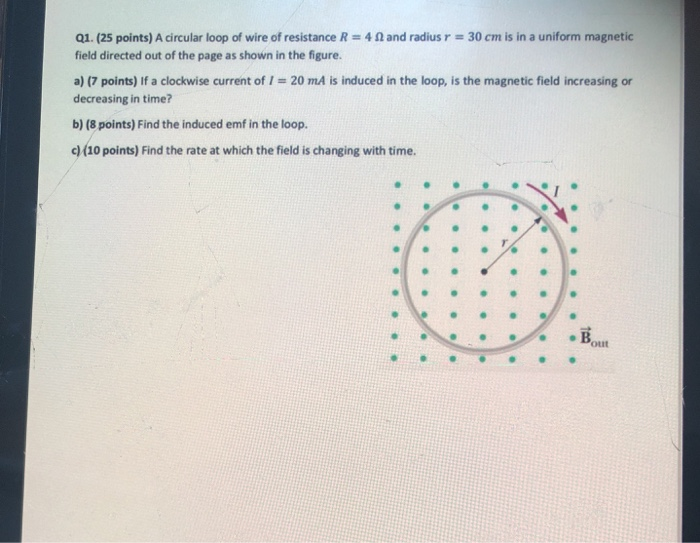Q1. (25 points) A circular loop of wire of resistance R = 4 N and radius r = 30 cm is in a uniform magnetic field directed out of the page as shown in the figure. a) (7 points) If a clockwise current of 1 = 20 mA is induced in the loop, is the magnetic field increasing or decreasing in time? b) (8 points) Find the induced emf in the loop. c) (10 points) Find the rate at which...

Free Homework App